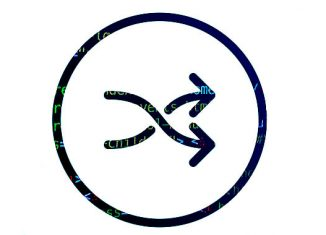# PHP shuffle an array with all values are in different positionHi all! PHP shuffle an array using shuffle(). But some times the values are in same position. The simple PHP script for shuffling an array with all values are in different position.

```function arrayshuffle(\$array_input)
{
\$array_shuffle=\$array_input;
while(count(array_intersect_assoc(\$array_input, \$array_shuffle))) {shuffle(\$array_shuffle); }
return \$array_shuffle;
}```

The sample Input and output as follow

```\$array_input=array(1,2,3,4,5);
print_r(\$array_input);
print_r(arrayshuffle(\$array_input));

Input
------
Array
(
 => 1
 => 2
 => 3
 => 4
 => 5
)

Output
------

Array
(
 => 3
 => 4
 => 2
 => 5
 => 1
)```